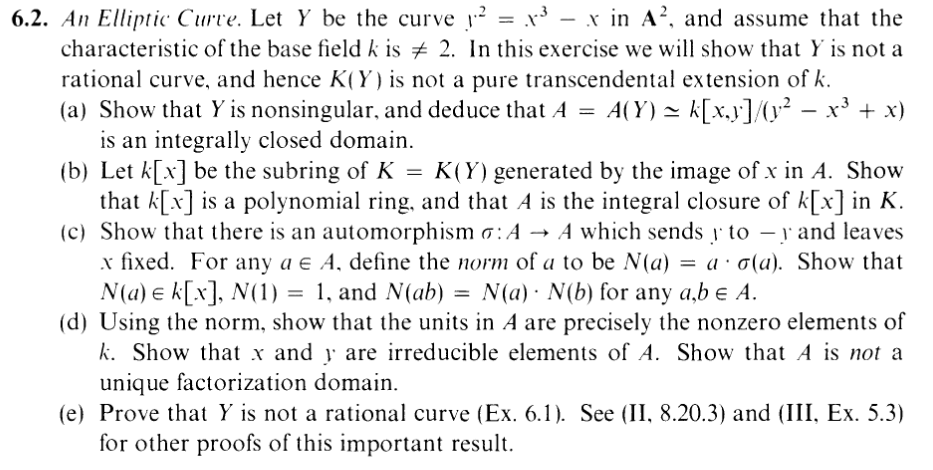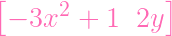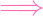I.6.2

9/2/2021### A

Have you ever wondered about elliptic curves? Elliptic curves show up in all sorts of applications, such as cryptography, and form the basis of many algebraic geometry problems. In particular, Section I.6.2 from Algebraic Geometry, by Robin Hartshorne explores this fascinating topic. It starts by considering whether a certain elliptic curve, given by y2 = x3 -x. The previous section of that book, section five, connects the concept of the Jacobian matrix with singularity. Therefore, if we take the Jacobian matrix, we obtain. Hence, a singular point would have to satisfy -3x2 + 1 = 0 as well as 2y = 0. Furthermore, in the case that chark = 3, then this is impossible. This is because this gives 1 = 0 on the first equation. On the other hand, if we assume chark2, 3 we obtain x2 = 13 and y = 0. Nevertheless, this point has to be on the original curve. The original curve equation is 0 = y2 - x3 + x. Therefore, if we plug in the identities, this reveals that 0 = 0 - (13)x + x. Furthermore, 0 = (23)x. Additionally, don't forget the assumption that chark2. This reveals that x = 0. However, this a contradiction of the statement that -3x2 + 1 = 0. Therefore, there are no singular points. Consequently, Y is nonsingular. Next, we have to conclude that A is integrally closed. One of the big lessons in section 6 of Hartshorne's work is that nonsingular points on curves have integrally closed local rings. According to Theorem I.3.2 (Hartshorne 17), A𝔪P is integrally closed when P is nonsingular. Furthermore, since every point is nonsingular, the localization is closed at every maximal ideal. However, according to Wikipedia ("Integrally closed domain"), this is equivalent to the fact that A itself is integrally closed. This fact begs the question: Is Y a rational curve? You might be wondering this. However, due to the fact that A is the integral closure of k[x] in K, the properties of the norm, and the fact that A is not a unique factorization domain, Y is not a rational curve.

### B

The first reason that Y is not a rational curve is that A is the integral closure of k[x] in K. In section six, during a proof of Lemma 6.5, Hartshorne states, "We consider the subring k[y] of K generated by y. Since k is algebraically closed, y is transcendental over k, hence k[y] is a polynomial ring" (41). This symbolizes the fact that k[x] is polynomial ring. This is because we can apply the same logic in our situation. Following this, we have to show that A is the integral closure of k[x] in K. In other words, if I is the integral closure of k[x], then we want to show that I = A. First, the inclusion I A is obvious. Furthermore, according to a solution online, "Since y2 k[x],y k[x]. So A k[x]" (Cutrone 24) Therefore, the reverse inclusion has been proven and this section is done.

### C

The next reason that Y is not rational is due to the properties of the norm. The exercise first asks us to "show that there is an automorphism σ : A A which sends y to -y and leaves x fixed" (Hartshorne 46). However, this is immediately obvious so there's nothing to do. On the other hand, the properties of the norm are more nontrivial to show. Let us start by showing N(a) k[x]. In other words, we want to get rid of the y terms, using the identity y2 = x3 - x. Let's say that a = iγixbiyci. Therefore, N(a) = a σ(a). Furthermore, N(a) = [ iγixbiyci][ iγjxbj(-y)cj], where i,j go over the same index set. Consequently, we can write N(a) as made up of terms in the form. L1 = γixbiyciγjxbj(-y)cj. This reveals that L2 = (-1)cjγ iγjxbi+bjyci+cj. In the case that c i + cj is even, this represents the fact that d : ci + cj = 2d. Therefore yci+cj = (y2)d. Furthermore, this is equal to (x3 - x)d. This gets rid of the y. So the even terms are safe. However, one may argue that ci + cj could be odd. To refute this point, let us assume without loss of generality that ci is odd and cj is even. In this situation, L1 turns into L1 = γiγjxbi+bjyci+cj. On the other hand, since i,j range over the same index set, there's another term "opposite" to L1, with i,j swapped. This term has the form L2 = γjxbjycjγ ixbi(-y)ci. Therefore, L2 = (-1)ciγiγjxbi+bjyci+cj. Furthermore, L 2 = -γiγjxbi+bjyci+cj. This follows from the fact that ci is odd. Thus, L1 and L2 cancel each other out. In total, we are left with a polynomial over x. This is equivalent to the statement that N(a) k[x]. The other two properties of N are obvious.

### D

Finally, Y is not a rational curve is due to the fact that A is not a unique factorization domain. This can be foreshadowed using a fact that I will call "Lemma One." The statement of this made-up Lemma reads, N(a) ka k. The proof of this Lemma is left to the reader. Now we get to the main point. If a comes from A, we would like to show that it is a unit if and only if it is a nonzero element of k. Lemma One reveals one side of this implication. It reveals the "if" side of the implication. Therefore, we need to show the "only if" side. Hence, let us suppose that a A is a unit. This happens if and only if there is an element b that comes from A such that ab = 1. This implies that N(ab) = 1. Therefore, N(a)N(b) = 1. Furthermore, N(a) k and N(a) k. Thus, a k, thanks to Lemma One. Next, we have to show that x and y are irreducible elements of A. First, let us suppose that x = ab. In that case, if we apply N to both sides, this reveals that N(x) = N(ab). Therefore, N(x) = N(a)N(b). Furthermore, since N(x) = x by definition, this reveals that x = N(a)N(b). Moreover, since k[x] is a polynomial ring, we are allowed to assume that either N(a) or N(b) is a unit. Finally, applying Lemma One completes the proof. Likewise, there is an analogous argument for y. Next, we have to show that A is not a unique factorization domain. This follows from the fact that we can write y2 as y y. However, we can also write it as x3 - x, which factors as x(x + 1)(x - 1). One of these factorizations has an irreducible factor of y. In contrast, the other has an irreducible factor of x. Hence, these are distinct factorizations of y2. Consequently, A is not a unique factorization domain.